# NCERT Solutions For Class 6 Maths Fractions Exercise 7.2

ncert textbook

## NCERT Solutions For Class 6 Maths Fractions Exercise 7.2

NCERT Solutions for Class 6 Maths Chapter 7 Fractions Ex 7.2

Exercise 7.2

Question 1.
Draw number lines and locate the points on them.Solution: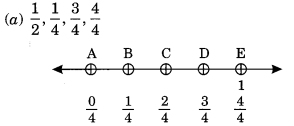We have divided the number line from 0 to 1 into four equal parts.
C represents$\frac { 2 }{ 4 }$i,e.,$\frac { 1 }{ 2 }$
B represents$\frac { 1 }{ 4 }$
D represents$\frac { 3 }{ 4 }$
and E represents$\frac { 4 }{ 4 }$, i.e., 1.We have divided the number line from 0 to 1 into eight equal parts.
B represents$\frac { 1 }{ 8 }$
C represents$\frac { 2 }{ 8 }$
D represents$\frac { 3 }{ 8 }$
and H represents$\frac { 7 }{ 8 }$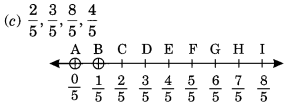From the above number line, we have
C represents$\frac { 2 }{ 5 }$
D represents$\frac { 3 }{ 5 }$
E represents$\frac { 4 }{ 5 }$
and I represents$\frac { 8 }{ 5 }$

Question 2.
Express the following as mixed fractions: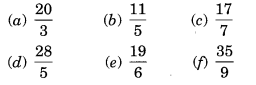Solution: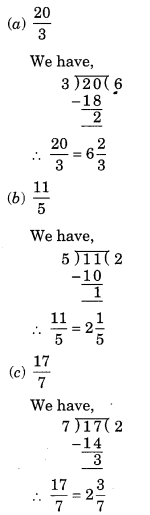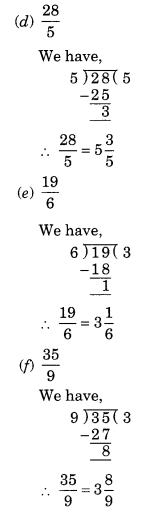Question 3.
Express the following as improper fractions: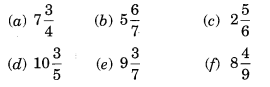Solution: